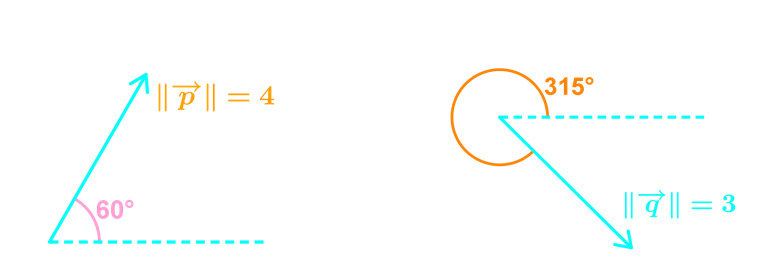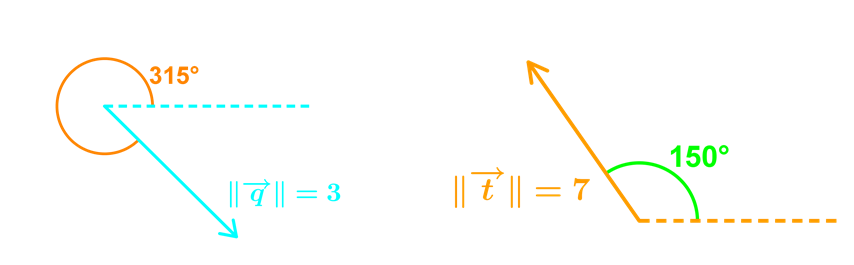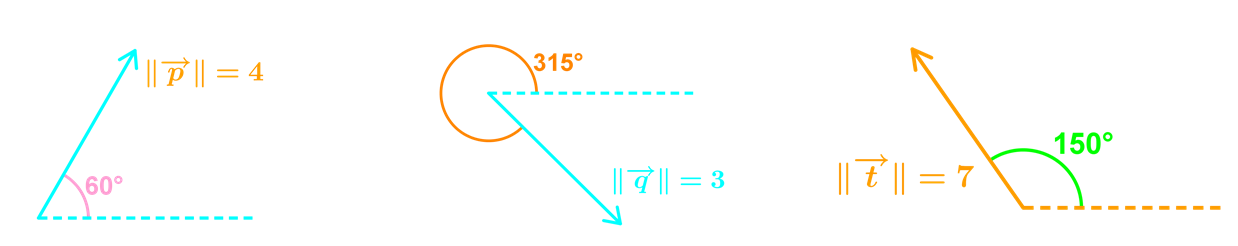# Operations on vectors in magnitude and direction form

### Operations on vectors in magnitude and direction form

By now we should be able to add or subtract vectors if we are given their components, but what if we are only given their magnitudes and direction angles instead? In this section, we will learn how to perform operations on vectors presented in magnitude and direction form, using the "three-steps approach".

#### Lessons

• Introduction

• 1.
Given the following vectors for $\vec{p}$, $\vec{q}$, $\vec{t}$find $\vec{p}+\vec{q}$

• 2.
Given the following vectors for $\vec{p}$, $\vec{q}$, $\vec{t}$find $\vec{t}+\vec{p}$

• 3.
Given the following vectors for $\vec{p}$, $\vec{q}$, $\vec{t}$find $\vec{p}+\vec{q}+\vec{t}$

• 4.
Particle $\beta$ is acted upon by the following forces:

• Force A with a magnitude of 30N towards north west
• Force B with a magnitude of 20N towards East
• Force C with a magnitude of 15N in the direction of 25° south of west
a)
find the magnitude of the resultant force

b)
find the direction of the resultant force